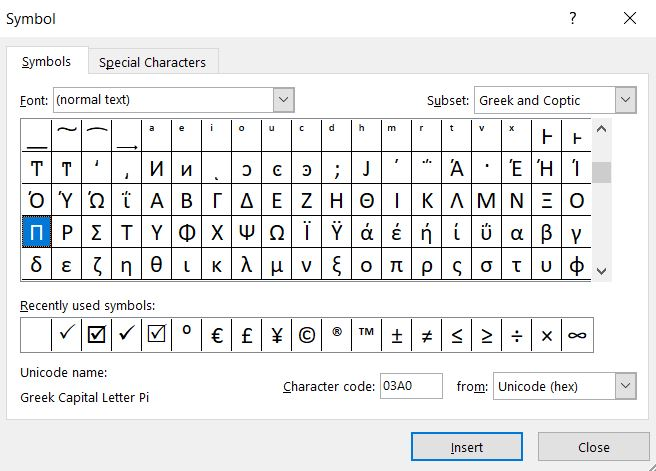### Pi in Excel

In this Excel article you will teach yourself how to use Pi in Excel.

The π is a special value known since ancient times. The pi value represents a half-rotation in the radian angle system.

### Pi function in Excel

Let’s start from using pi in Excel. Syntax of PI function is very easy. There are no arguments of pi function. Excel PI formula is just =PI()

Excel Pi function returns pi value up to 15 digits.

π in Excel equals exactly 3.14159265358979

### How to type pi in Excel?

You can insert π symbol in Excel. To do that go to Insert tab and click Symbol button.Greek capital letter Pi you can find in Greek and Coptic subset.

### Example of using pi in Excel

You can use pi function to calculate area of the circle. Use this pi Excel formula

=PI()*A2^2## Template

```Further reading: